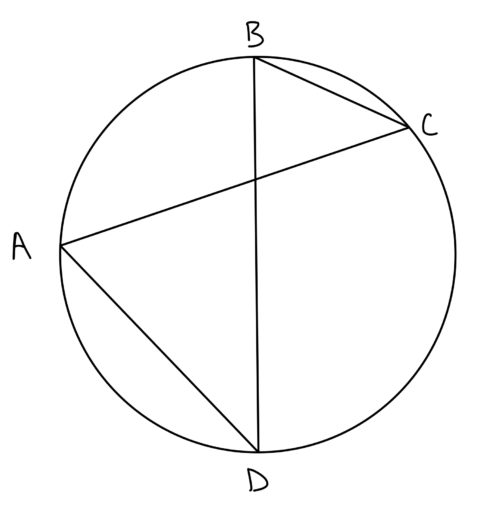There are also two relationships between angles and circles which are crucial. First, let's define the term "central angle":

Central Angle: A central angle is an angle located at the center of a circle with endpoints on the circumference of that circle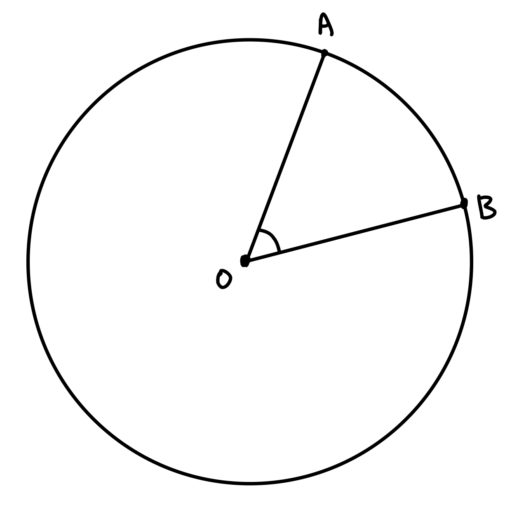Now, the crucial fact to learn is:

Central Angles and Arcs: In the following diagram: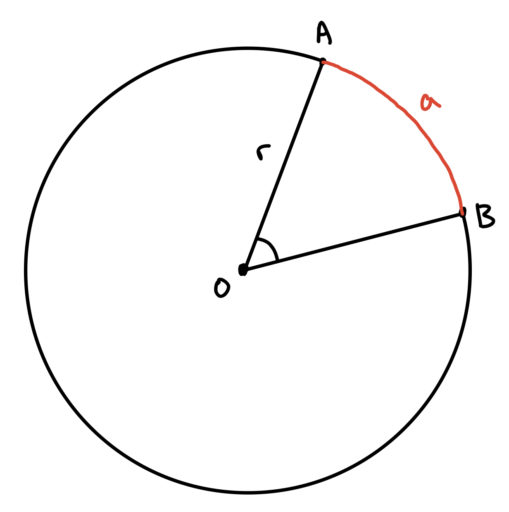The degree of angle AOB =Thus, we can determine the degree of any central angle by looking at the length of its arc, and vice versa.

Example 1
The circle below has a radius of 2 andhas a length of. What is the degree of angle?Example 2
The circle below has a radius of 6 and the degree ofis 47 degrees. What is the length of?A related fact to the above is:

Circumference Angle Theorem: In the following circle with radius: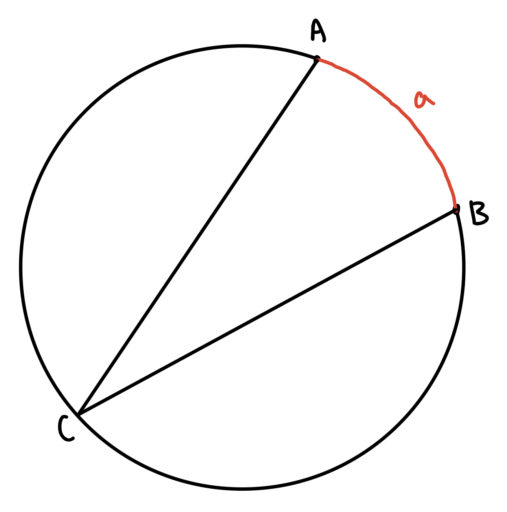The degree of angle ACB =.

This theorem is also called the Inscribed Angle Theorem.

In essence, if you have an angle on the circumference of a circle, its degree will be one-half the degree of a central angle with the same endpoints. In pictures: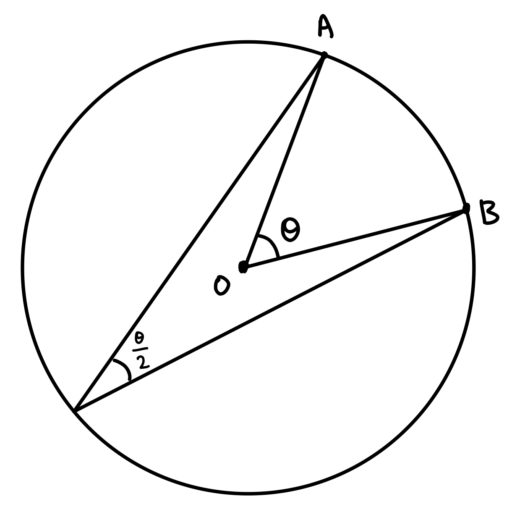Now, what may be surprising about this is that it does matter where on the circumference the angle begins from. In other words: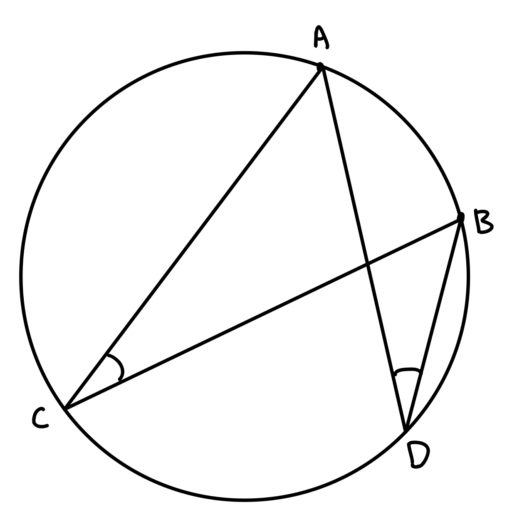the anglesandabove have the same degree.

Finally, you must be careful to make sure that you are comparing the angle on the circumference to the correct central angle. For example: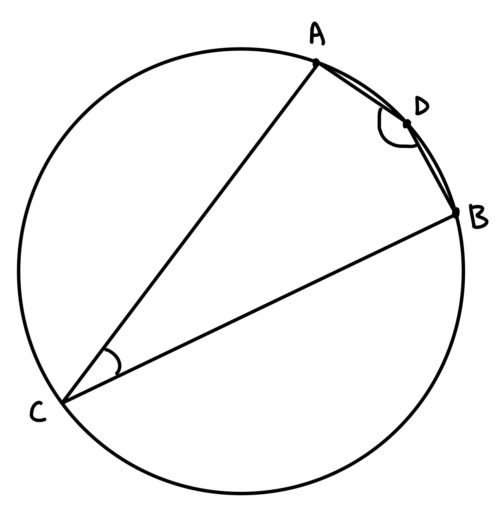anddo not have the same degree. That is because they give rise to different central angles: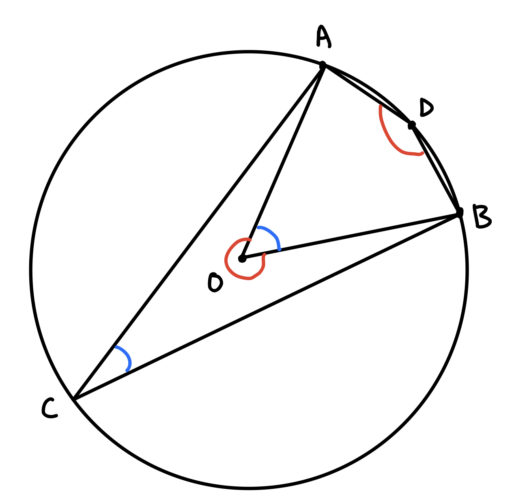Thus, it is not enough to look at the endpoints of your angle. You also need to look at where on the circumference the angle is, so you can be sure to compare it to the relevant central angle.

Practice Problems
1. The below circle has a center at. Find: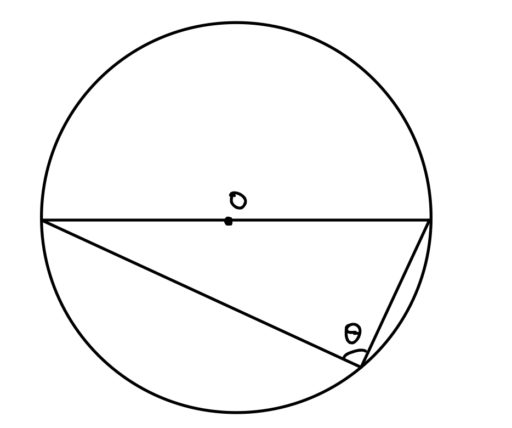2. Find the value of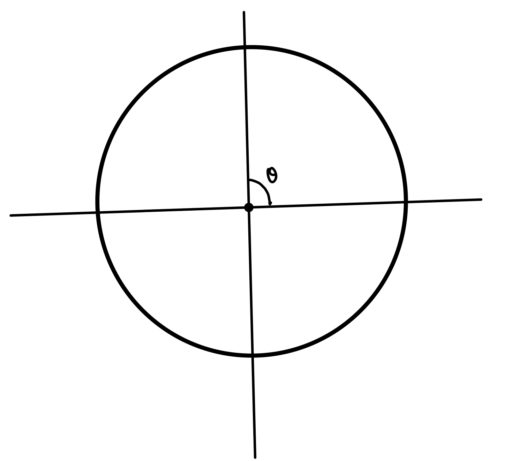3. In the following diagram,Find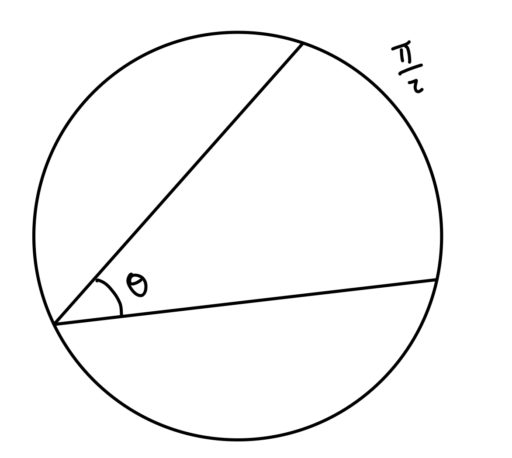4. The radius of the following circle isFind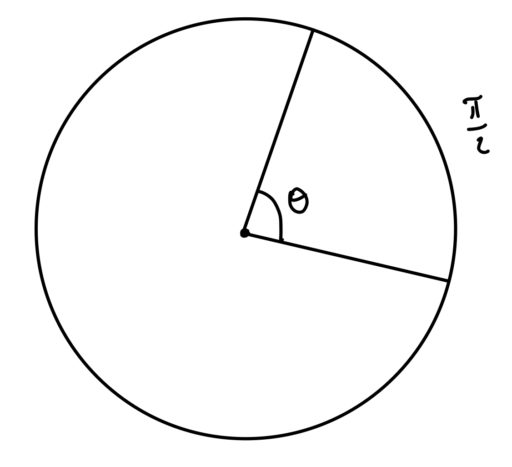5. The radius of the following circle is 1. Find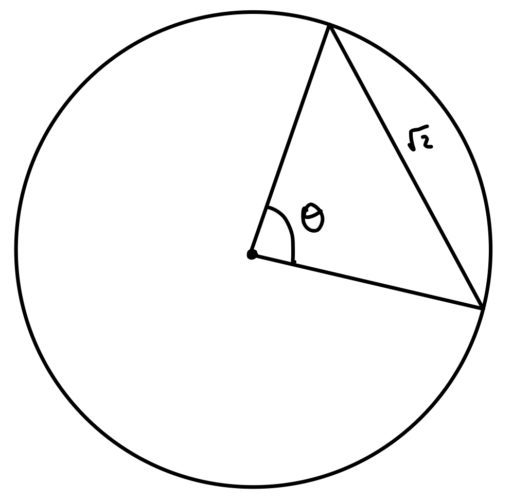6. The radius of the below circle is 1. Find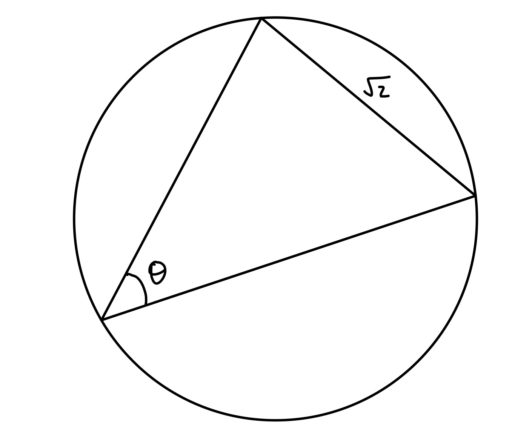7. In the below circle, which is larger?A.B.C. They are equal.
D. It cannot be determined.